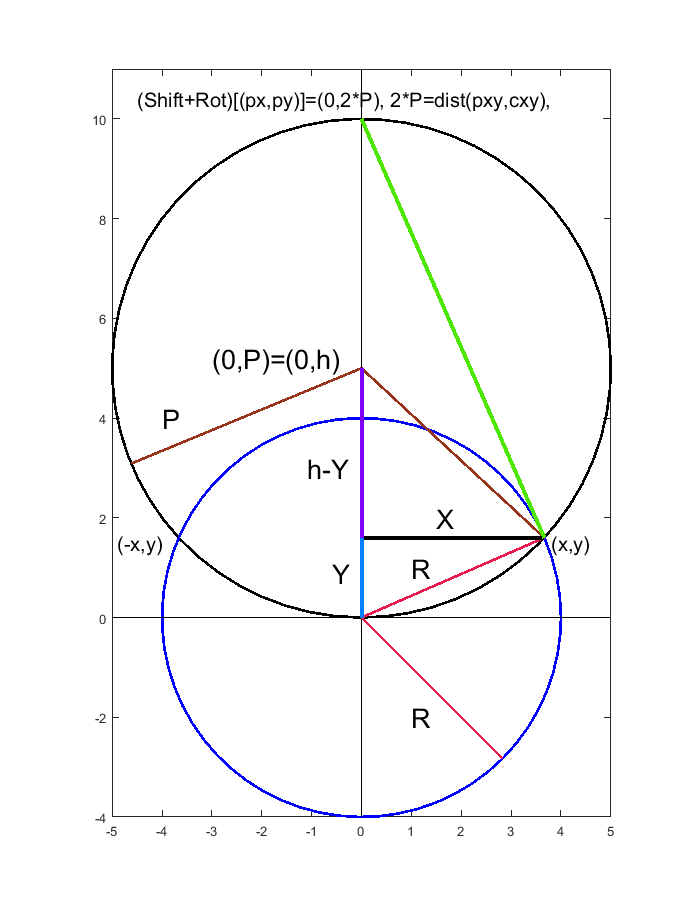# Problem 58872. Find the Points Tangent to a Circle from an External Point

From a point where do the lines touch a circle tangentially?. The loldrup solution may provide some guidance and alternate method. I will elaborate a more reference frame modification geometric solution utilizing Matlab specific functions, rotation matrix, and translation matrix.
Given point(px,py) and circle [cx,cy,R] return the circle points [x3 y3;x4 y4] where lines thru the point are tangential to the circle. The line ([px,py],[x3,y3]) is tangential to circle [cx,cy,R] at circle point [x3,y3]. D>R.
The below figure is created based upon h=P=distance([cx,cy],[px,py])/2=D/2, translating (cx,cy) to (0,0), and rotating (px,py) to be on the Y-axis. From this manipulation two right triangles are apparent: [X,Y,R] and [X,h-Y,P]. Subtracting and simplifying these triangles leads to Y and two X values after substituting back into R^2=X^+Y^2 equation.
P^2=X^2+(h-Y)^2 and R^2=X^2+Y^2 after subtraction gives R^2-P^2=Y^2-(h-Y)^2 = Y^2-h^2+2hY-Y^2=2hY-h^2 thus
Y=(R^2-P^2+h^2)/(2h) =(R^2-P^2+P^2)/(2P)=R^2/(2P)=R^2/D
X=+/- (R^2-Y^2)^.5=+/- (R^2-(R^2/(2P))^2)^0.5=+/- R*(1-(R/D)^2)^0.5
The trick is to now un-rotate and translate this solution matrix using t=atan2(dx,dy), [cos(t) -sin(t);sin(t) cos(t)] and [cx cy]This figure shows the point (px,py) rotated onto the Y-axis at position 2P. The circle (cx,cy) has been shifted to the origin with radius R. The green line shows a tangent at (x,y) from (px,py). A second tangent point is at (-x.y). D=2*P

### Solution Stats

100.0% Correct | 0.0% Incorrect
Last Solution submitted on Aug 29, 2023

### Community Treasure Hunt

Find the treasures in MATLAB Central and discover how the community can help you!

Start Hunting!